# kmed: Distance-Based K-Medoids

#### 2022-08-29

Abstract

The kmed vignette consists of four sequantial parts of distance-based (k-medoids) cluster analysis. The first part is defining the distance. It has numerical, binary, categorical, and mixed distances. The next part is applying a clustering algorithm in the pre-defined distance. There are five k-medoids presented, namely the simple and fast k-medoids, k-medoids, ranked k-medoids, increasing number of clusters in k-medoids, and simple k-medoids. After the clustering result is obtained, a validation step is required. The cluster validation applies internal and relative criteria. The last part is visualizing the cluster result in a biplot or marked barplot.

## 1. Introduction

The kmed package is designed to analyse k-medoids based clustering. The features include:

• distance computation:
• numerical variables:
• binary or categorical variables:
• mixed variables:
• k-medoids algorithms:
• cluster validations:
• Cluster visualizations:

## 2. Distance Computation

### 2.A. Numerical variables (distNumeric)

The distNumeric function can be applied to calculate numerical distances. There are four distance options, namely Manhattan weighted by range (mrw), squared Euclidean weighted by range (ser), squared Euclidean weighted by squared range (ser.2), squared Euclidean weighted by variance (sev), and unweighted squared Euclidean (se). The distNumeric function provides method in which the desired distance method can be selected. The default method is mrw.

The distance computation in a numerical variable data set is performed in the iris data set. An example of manual calculation of the numerical distances is applied for the first and second objects only to introduce what the distNumeric function does.

library(kmed)
## Warning: replacing previous import 'lifecycle::last_warnings' by
## 'rlang::last_warnings' when loading 'pillar'
iris[1:3,]
##   Sepal.Length Sepal.Width Petal.Length Petal.Width Species
## 1          5.1         3.5          1.4         0.2  setosa
## 2          4.9         3.0          1.4         0.2  setosa
## 3          4.7         3.2          1.3         0.2  setosa

#### 2.A.1. Manhattan weighted by range (method = "mrw")

By applying the distNumeric function with method = "mrw", the distance among objects in the iris data set can be obtained.

num <- as.matrix(iris[,1:4])
rownames(num) <- rownames(iris)
#calculate the Manhattan weighted by range distance of all iris objects
mrwdist <- distNumeric(num, num)
#show the distance among objects 1 to 3
mrwdist[1:3,1:3]
##           1         2         3
## 1 0.0000000 0.2638889 0.2530603
## 2 0.2638889 0.0000000 0.1558380
## 3 0.2530603 0.1558380 0.0000000

The Manhattan weighted by range distance between objects 1 and 2 is 0.2638889. To calculate this distance, the range of each variable is computed.

#extract the range of each variable
apply(num, 2, function(x) max(x)-min(x))
## Sepal.Length  Sepal.Width Petal.Length  Petal.Width
##          3.6          2.4          5.9          2.4

Then, the distance between objects 1 and 2 is

#the distance between objects 1 and 2
abs(5.1-4.9)/3.6 + abs(3.5 - 3.0)/2.4 + abs(1.4-1.4)/5.9 + abs(0.2-0.2)/2.4
##  0.2638889

which is based on the data

##   Sepal.Length Sepal.Width Petal.Length Petal.Width
## 1          5.1         3.5          1.4         0.2
## 2          4.9         3.0          1.4         0.2

(Back to Intoduction)

#### 2.A.2. squared Euclidean weighted by range (method = "ser")

#calculate the squared Euclidean weighthed by range distance of all iris objects
serdist <- distNumeric(num, num, method = "ser")
#show the distance among objects 1 to 3
serdist[1:3,1:3]
##            1          2          3
## 1 0.00000000 0.11527778 0.08363936
## 2 0.11527778 0.00000000 0.02947269
## 3 0.08363936 0.02947269 0.00000000

The squared Euclidean weighted by range distance between objects 1 and 2 is 0.11527778. It is obtained by

#the distance between objects 1 and 2
(5.1-4.9)^2/3.6 + (3.5 - 3.0)^2/2.4 + (1.4-1.4)^2/5.9 + (0.2-0.2)^2/2.4
##  0.1152778

(Back to Intoduction)

#### 2.A.3. squared Euclidean weighted by squared range (method = "ser.2")

#calculate the squared Euclidean weighthed by squared range distance of
#all iris objects
ser.2dist <- distNumeric(num, num, method = "ser.2")
#show the distance among objects 1 to 3
ser.2dist[1:3,1:3]
##            1          2          3
## 1 0.00000000 0.04648920 0.02825795
## 2 0.04648920 0.00000000 0.01031814
## 3 0.02825795 0.01031814 0.00000000

The squared Euclidean weighted by squared range distance between objects 1 and 2 is 0.04648920 that is computed by

(5.1-4.9)^2/3.6^2 + (3.5 - 3.0)^2/2.4^2 + (1.4-1.4)^2/5.9^2 + (0.2-0.2)^2/2.4^2
##  0.0464892

where the data are

##   Sepal.Length Sepal.Width Petal.Length Petal.Width
## 1          5.1         3.5          1.4         0.2
## 2          4.9         3.0          1.4         0.2

(Back to Intoduction)

#### 2.A.4. squared Euclidean weighted by variance (method = "sev")

#calculate the squared Euclidean weighthed by variance distance of
#all iris objects
sevdist <- distNumeric(num, num, method = "sev")
#show the distance among objects 1 to 3
sevdist[1:3,1:3]
##           1         2         3
## 1 0.0000000 1.3742671 0.7102849
## 2 1.3742671 0.0000000 0.2720932
## 3 0.7102849 0.2720932 0.0000000

The squared Euclidean weighted by variance distance between objects 1 and 2 is 1.3742671. To compute this distance, the variance of each variable is calculated.

#calculate the range of each variable
apply(num[,1:4], 2, function(x) var(x))
## Sepal.Length  Sepal.Width Petal.Length  Petal.Width
##    0.6856935    0.1899794    3.1162779    0.5810063

Then, the distance between objects 1 and 2 is

(5.1-4.9)^2/0.6856935 + (3.5 - 3.0)^2/0.1899794 + (1.4-1.4)^2/3.1162779 +
(0.2-0.2)^2/0.5810063
##  1.374267

(Back to Intoduction)

#### 2.A.5. squared Euclidean (method = "se")

#calculate the squared Euclidean distance of all iris objects
sedist <- distNumeric(num, num, method = "se")
#show the distance among objects 1 to 3
sedist[1:3,1:3]
##      1    2    3
## 1 0.00 0.29 0.26
## 2 0.29 0.00 0.09
## 3 0.26 0.09 0.00

The squared Euclidean distance between objects 1 and 2 is 0.29. It is computed by

(5.1-4.9)^2 + (3.5 - 3.0)^2 + (1.4-1.4)^2 + (0.2-0.2)^2
##  0.29

(Back to Intoduction)

### 2.B. Binary or Categorical variables

There are two functions to calculate the binary and categorical variables. The first is matching to compute the simple matching distance and the second is cooccur to calculate the co-occurrence distance. To introduce what these functions do, the bin data set is generated.

set.seed(1)
bin <- matrix(sample(1:2, 4*2, replace = TRUE), 4, 2)
rownames(bin) <- 1:nrow(bin)
colnames(bin) <- c("x", "y")

#### 2.B.1. Simple matching (matching)

The matching function calculates the simple matching distance between two data sets. If the two data sets are identical, the functions calculates the distance among objects within the data set. The simple matching distance is equal to the proportion of the mis-match categories.

bin
##   x y
## 1 1 2
## 2 2 1
## 3 1 1
## 4 1 1
#calculate simple matching distance
matching(bin, bin)
##     1   2   3   4
## 1 0.0 1.0 0.5 0.5
## 2 1.0 0.0 0.5 0.5
## 3 0.5 0.5 0.0 0.0
## 4 0.5 0.5 0.0 0.0

As an example of the simple matching distance, the distance between objects 1 and 2 is calculated by

((1 == 1) + (1 == 2))/ 2
##  0.5

The distance between objects 1 and 2, which is 0.5, is produced from one mis-match and one match categories from the two variables (x and y) in the bin data set. When x1 is equal to x2, for instance, the score is 0. Meanwile, if x1 is not equal to x2, the score is 1. These scores are also valid in the y variable. Hence, the distance between objects 1 and 2 is (0+1)/2 that is equal to 1/2.

(Back to Intoduction)

#### 2.B.2. Co-occurrence distance (cooccur)

The co-ocurrence distance (Ahmad and Dey 2007; Harikumar and PV 2015) can be calculated via the cooccur function. To calculate the distance between objects, the distribution of the variables are taken into consideration. Compared to the simple matching distance, the co-occurrence distance redefines the score of match and mis-match categories such that they are unnecessary to be 0 and 1, respectively. Due to relying on the distribution of all inclusion variables, the co-occurence distance of a data set with a single variable is absent.

The co-occurrence distance of the bin data set is

#calculate co-occurrence distance
cooccur(bin)
##           1         2         3         4
## 1 0.0000000 0.6666667 0.3333333 0.3333333
## 2 0.6666667 0.0000000 0.3333333 0.3333333
## 3 0.3333333 0.3333333 0.0000000 0.0000000
## 4 0.3333333 0.3333333 0.0000000 0.0000000

To show how co-occurrence distance is calculated, the distance between objects 1 and 2 is presented.

bin
##   x y
## 1 1 2
## 2 2 1
## 3 1 1
## 4 1 1

Step 1 Creating cross tabulations

#cross tabulation to define score in the y variable
(tab.y <- table(bin[,'x'], bin[,'y']))
##
##     1 2
##   1 2 1
##   2 1 0
#cross tabulation to define score in the x variable
(tab.x <- table(bin[,'y'], bin[,'x']))
##
##     1 2
##   1 2 1
##   2 1 0

Step 2 Calculating the column proportions of each cross tabulation

#proportion in the y variable
(prop.y <- apply(tab.y, 2, function(x) x/sum(x)))
##
##             1 2
##   1 0.6666667 1
##   2 0.3333333 0
#proportion in the x variable
(prop.x <- apply(tab.x, 2, function(x) x/sum(x)))
##
##             1 2
##   1 0.6666667 1
##   2 0.3333333 0

Step 3 Finding the maximum values for each row of the proportion

#maximum proportion in the y variable
(max.y <- apply(prop.y, 2, function(x) max(x)))
##         1         2
## 0.6666667 1.0000000
#maximum proportion in the x variable
(max.x <- apply(prop.x, 2, function(x) max(x)))
##         1         2
## 0.6666667 1.0000000

Step 4 Defining the scores of each variable

The score is obtained by a summation of the maximum value subtracted and divided by a constant. The constant has a value depending on the number of inclusion variables. For the bin data set, the constant is 1 because both x and y variables are only depended on one other variable, i.e. x depends on the distribution of y and y relies on the distribution of x.

#score mis-match in the y variable
(sum(max.y) - 1)/1
##  0.6666667
#score mis-match in the x variable
(sum(max.x) - 1)/1
##  0.6666667

It can be implied that the score for mis-match categories are 0.5 and 0.67 in the x and y variables, respectively. Note that the score for match categories is alwalys 0. Thus, the distance between objects 1 and 2 is 0+0.6666667 = 0.6666667 and between objects 1 and 3 is 0.5+0.6666667 = 1.1666667

(Back to Intoduction)

### 2.C. Mixed variables (distmix)

There are six available distance methods for a mixed variable data set. The distmix function calculates mixed variable distance in which it requires column id of each class of variables. The mixdata data set is generated to describe each method in the distmix function.

cat <- matrix(c(1, 3, 2, 1, 3, 1, 2, 2), 4, 2)
mixdata <- cbind(iris[c(1:2, 51:52),3:4], bin, cat)
rownames(mixdata) <- 1:nrow(mixdata)
colnames(mixdata) <- c(paste(c("num"), 1:2, sep = ""),
paste(c("bin"), 1:2, sep = ""),
paste(c("cat"), 1:2, sep = ""))

#### 2.C.1 Gower (method = "gower")

The method = "gower" in the distmix function calculates the Gower (1971) distance. The original Gower distance allows missing values, while it is not allowed in the distmix function.

mixdata
##   num1 num2 bin1 bin2 cat1 cat2
## 1  1.4  0.2    1    2    1    3
## 2  1.4  0.2    2    1    3    1
## 3  4.7  1.4    1    1    2    2
## 4  4.5  1.5    1    1    1    2

The Gower distance of the mixdata data set is

#calculate the Gower distance
distmix(mixdata, method = "gower", idnum = 1:2, idbin = 3:4, idcat = 5:6)
##           1         2         3         4
## 1 0.0000000 0.6666667 0.8205128 0.6565657
## 2 0.6666667 0.0000000 0.8205128 0.8232323
## 3 0.8205128 0.8205128 0.0000000 0.1895882
## 4 0.6565657 0.8232323 0.1895882 0.0000000

As an example, the distance between objects 3 and 4 is presented. The range of each numerical variables is necessary.

#extract the range of each numerical variable
apply(mixdata[,1:2], 2, function(x) max(x)-min(x))
## num1 num2
##  3.3  1.3

The Gower distance calculates the Gower similarity first. In the Gower similarity, the mis-match categories in the binary/ categorical variables are scored 0 and the match categories are 1. Meanwhile, in the numerical variables, 1 is subtracted by a ratio between the absolute difference and its range. Then, the Gower similarity can be weighted by the number of variables. Thus, the Gower similarity between objects 3 and 4 is

#the Gower similarity
(gowsim <- ((1-abs(4.7-4.5)/3.3) + (1-abs(1.4-1.5)/1.3) + 1 + 1 + 0 + 1)/ 6 ) 
##  0.8104118

The Gower distance is obtained by subtracting 1 with the Gower similarity. The distance between objects 3 and 4 is then

#the Gower distance
1 - gowsim
##  0.1895882

(Back to Intoduction)

#### 2.C.2 Wishart (method = "wishart")

The Wishart (2003) distance can be calculated via method = "wishart". Although it allows missing values, it is again illegitimate in the distmix function. The Wishart distance for the mixdata is

#calculate the Wishart distance
distmix(mixdata, method = "wishart", idnum = 1:2, idbin = 3:4, idcat = 5:6)
##           1         2         3         4
## 1 0.0000000 0.8164966 1.2206686 1.1578998
## 2 0.8164966 0.0000000 1.2206686 1.2277616
## 3 1.2206686 1.2206686 0.0000000 0.4144946
## 4 1.1578998 1.2277616 0.4144946 0.0000000

To calculate the Wishart distance, the variance of each numerical variable is required. It weighs the squared difference of a numerical variable.

#extract the variance of each numerical variable
apply(mixdata[,1:2], 2, function(x) var(x))
##   num1   num2
## 3.4200 0.5225

Meanwhile, the mis-match categories in the binary/ categorical variables are scored 1 and the match categories are 0. Then, all score of the variables is added and squared rooted. Thus, the distance between objects 3 and 4 is

wish <- (((4.7-4.5)^2/3.42) + ((1.4-1.5)^2/0.5225) + 0 + 0 + 1 + 0)/ 6
#the Wishart distance
sqrt(wish)
##  0.4144946

(Back to Intoduction)

#### 2.C.3 Podani (method = "podani")

The method = "podani" in the distmix function calculates the Podani (1999) distance. Similar to The Gower and Wishart distances, it allows missing values, yet it is not allowed in the distmix function. The Podani distance for the mixdata is

#calculate Podani distance
distmix(mixdata, method = "podani", idnum = 1:2, idbin = 3:4, idcat = 5:6)
##          1        2        3        4
## 1 0.000000 2.000000 2.202742 1.970396
## 2 2.000000 0.000000 2.202742 2.209629
## 3 2.202742 2.202742 0.000000 1.004784
## 4 1.970396 2.209629 1.004784 0.000000

The Podani and Wishart distances are similar. They are different in the denumerator for the numerical variables. Instead of a variance, the Podani distance applies the squared range for a numerical variable. Unlike the Gower and Podani distances, the number of variables as a weight is absent in the Podani distance. Hence, the distance between objects 3 and 4 is

poda <- ((4.7-4.5)^2/3.3^2) + ((1.4-1.5)^2/1.3^2) + 0 + 0 + 1 + 0
#the Podani distance
sqrt(poda)
##  1.004784

which is based on data

##   num1 num2 bin1 bin2 cat1 cat2
## 3  4.7  1.4    1    1    2    2
## 4  4.5  1.5    1    1    1    2

(Back to Intoduction)

#### 2.C.4 Huang (method = "huang")

The method = "huang" in the distmix function calculates the Huang (1997) distance. The Huang distance of the mixdata data set is

#calculate the Huang distance
distmix(mixdata, method = "huang", idnum = 1:2, idbin = 3:4, idcat = 5:6)
##           1         2         3         4
## 1  0.000000  5.144332 16.188249 13.872166
## 2  5.144332  0.000000 16.188249 15.158249
## 3 16.188249 16.188249  0.000000  1.336083
## 4 13.872166 15.158249  1.336083  0.000000

The average standard deviation of the numerical variables is required to calculate the Huang distance. This measure weighs the binary/ categorical variables.

#find the average standard deviation of the numerical variables
mean(apply(mixdata[,1:2], 2, function(x) sd(x)))
##  1.286083

While the squared difference of the numerical variables is calculated, the mis-match categories are scored 1 and the match categories are 0 in the binary/ categorical variables. Thus, the distance between objects 3 and 4 is

(4.7-4.5)^2 + (1.4-1.5)^2 + 1.286083*(0 + 0) + 1.286083*(1 + 0)
##  1.336083

(Back to Intoduction)

#### 2.C.5 Harikumar and PV (method = "harikumar")

The Harikumar and PV (2015) distance can be calculated via method = "harikumar". The Harikumar and PV distance for the mixdata is

#calculate Harikumar-PV distance
distmix(mixdata, method = "harikumar", idnum = 1:2, idbin = 3:4, idcat = 5:6)
##     1   2   3   4
## 1 0.0 4.0 6.5 5.9
## 2 4.0 0.0 7.5 7.4
## 3 6.5 7.5 0.0 0.8
## 4 5.9 7.4 0.8 0.0

The Harikumar and PV distance requires an absolute difference in the numerical variables and unweighted simple matching, i.e. Hamming distance, in the binary variables. For the categorical variables, it applies co-occurrence distance. The co-occurence distance in the categorical variables is (for manual calculation see co-occurrence subsection)

cooccur(mixdata[,5:6])
##     1 2   3   4
## 1 0.0 2 1.0 0.5
## 2 2.0 0 2.0 2.0
## 3 1.0 2 0.0 0.5
## 4 0.5 2 0.5 0.0

Hence, the distance between objects 1 and 3 is

abs(4.7-4.5) + abs(1.4-1.5) + (0 + 0) + (0.5)
##  0.8

where the data are

##   num1 num2 bin1 bin2 cat1 cat2
## 3  4.7  1.4    1    1    2    2
## 4  4.5  1.5    1    1    1    2

(Back to Intoduction)

#### 2.C.6 Ahmad and Dey (method = "ahmad")

The method = "ahmad" in the distmix function calculates the Ahmad and Dey (2007) distance. The Ahmad and Dey distance of the mixdata data set is

#calculate Ahmad-Dey distance
distmix(mixdata, method = "ahmad", idnum = 1:2, idbin = 3:4, idcat = 5:6)
##          1        2          3          4
## 1  0.00000 10.74383 14.5800000 12.6611111
## 2 10.74383  0.00000 16.7867901 16.4882716
## 3 14.58000 16.78679  0.0000000  0.1611111
## 4 12.66111 16.48827  0.1611111  0.0000000

The Ahmad and dey distance requires a squared difference in the numerical variables and co-occurrence distance for both the binary and categorical variables. The co-occurrence distance in the mixdata data set is

cooccur(mixdata[,3:6])
##          1        2         3         4
## 1 0.000000 3.277778 1.5000000 1.1666667
## 2 3.277778 0.000000 2.1111111 2.2777778
## 3 1.500000 2.111111 0.0000000 0.3333333
## 4 1.166667 2.277778 0.3333333 0.0000000

Thus, the distance between objects 2 and 3 is

(1.4-4.7)^2 + (0.2-1.4)^2 + (2)^2
##  16.33

which is based on the data

##   num1 num2 bin1 bin2 cat1 cat2
## 2  1.4  0.2    2    1    3    1
## 3  4.7  1.4    1    1    2    2

(Back to Intoduction)

## 3. K-medoids algorithms

There are some k-medoids algorithms available in this package. They are the simple and fast k-medoids (fastkmed), k-medoids, ranked k-medoids (rankkmed), and increasing number of clusters k-medoids (inckmed). All algorithms have a list of results, namely the cluster membership, id medoids, and distance of all objects to their medoid.

In this section, the algorithms are applied in the iris data set by applying the mrw distance (see Manhattan weighted by range). The number of clusters in this data set is 3.

### 3.A. Simple and fast k-medoids algorithm (fastkmed)

The simple and fast k-medoid (SFKM) algorithm has been proposed by Park and Jun (2009). The fastkmed function runs this algorithm to cluster the objects. The compulsory inputs are a distance matrix or distance object and a number of clusters. Hence, the SFKM algorithm for the iris data set is

#run the sfkm algorihtm on iris data set with mrw distance
(sfkm <- fastkmed(mrwdist, ncluster = 3, iterate = 50))
## $cluster ## 1 2 3 4 5 6 7 8 9 10 11 12 13 14 15 16 17 18 19 20 ## 1 1 1 1 1 1 1 1 1 1 1 1 1 1 1 1 1 1 1 1 ## 21 22 23 24 25 26 27 28 29 30 31 32 33 34 35 36 37 38 39 40 ## 1 1 1 1 1 1 1 1 1 1 1 1 1 1 1 1 1 1 1 1 ## 41 42 43 44 45 46 47 48 49 50 51 52 53 54 55 56 57 58 59 60 ## 1 1 1 1 1 1 1 1 1 1 3 3 3 2 3 2 3 2 2 2 ## 61 62 63 64 65 66 67 68 69 70 71 72 73 74 75 76 77 78 79 80 ## 2 2 2 2 2 3 2 2 2 2 3 2 2 2 2 3 3 3 2 2 ## 81 82 83 84 85 86 87 88 89 90 91 92 93 94 95 96 97 98 99 100 ## 2 2 2 2 2 2 3 2 2 2 2 2 2 2 2 2 2 2 2 2 ## 101 102 103 104 105 106 107 108 109 110 111 112 113 114 115 116 117 118 119 120 ## 3 3 3 3 3 3 2 3 3 3 3 3 3 3 3 3 3 3 3 2 ## 121 122 123 124 125 126 127 128 129 130 131 132 133 134 135 136 137 138 139 140 ## 3 3 3 3 3 3 3 3 3 3 3 3 3 3 2 3 3 3 3 3 ## 141 142 143 144 145 146 147 148 149 150 ## 3 3 3 3 3 3 3 3 3 3 ## ##$medoid
##    8  95 148
##
## $minimum_distance ##  45.76718 Then, a classification table can be obtained. (sfkmtable <- table(sfkm$cluster, iris[,5]))
##
##     setosa versicolor virginica
##   1     50          0         0
##   2      0         39         3
##   3      0         11        47

Applying the SFKM algorithm in iris data set with the Manhattan weighted by range, the misclassification rate is

(3+11)/sum(sfkmtable)
##  0.09333333

(Back to Intoduction)

### 3.B. K-medoids algorithm

Reynolds et al. (2006) has been proposed a k-medoids (KM) algorithm. It is similar to the SFKM such that the fastkmed can be applied. The difference is in the initial medoid selection where the KM selects the initial medoid randomly. Thus, the KM algorithm for the iris data set by setting the init is

#set the initial medoids
set.seed(1)
(kminit <- sample(1:nrow(iris), 3))
##   68 129  43
#run the km algorihtm on iris data set with mrw distance
(km <- fastkmed(mrwdist, ncluster = 3, iterate = 50, init = kminit))
## $cluster ## 1 2 3 4 5 6 7 8 9 10 11 12 13 14 15 16 17 18 19 20 ## 3 3 3 3 3 3 3 3 3 3 3 3 3 3 3 3 3 3 3 3 ## 21 22 23 24 25 26 27 28 29 30 31 32 33 34 35 36 37 38 39 40 ## 3 3 3 3 3 3 3 3 3 3 3 3 3 3 3 3 3 3 3 3 ## 41 42 43 44 45 46 47 48 49 50 51 52 53 54 55 56 57 58 59 60 ## 3 3 3 3 3 3 3 3 3 3 2 2 2 1 1 1 2 1 1 1 ## 61 62 63 64 65 66 67 68 69 70 71 72 73 74 75 76 77 78 79 80 ## 1 1 1 1 1 2 1 1 1 1 2 1 1 1 1 2 1 2 1 1 ## 81 82 83 84 85 86 87 88 89 90 91 92 93 94 95 96 97 98 99 100 ## 1 1 1 1 1 1 2 1 1 1 1 1 1 1 1 1 1 1 1 1 ## 101 102 103 104 105 106 107 108 109 110 111 112 113 114 115 116 117 118 119 120 ## 2 2 2 2 2 2 1 2 2 2 2 2 2 2 2 2 2 2 2 1 ## 121 122 123 124 125 126 127 128 129 130 131 132 133 134 135 136 137 138 139 140 ## 2 2 2 2 2 2 2 2 2 2 2 2 2 2 1 2 2 2 2 2 ## 141 142 143 144 145 146 147 148 149 150 ## 2 2 2 2 2 2 2 2 2 2 ## ##$medoid
##  100 148   8
##
## $minimum_distance ##  48.8411 The classification table of the KM algorithm is (kmtable <- table(km$cluster, iris[,5]))
##
##     setosa versicolor virginica
##   1      0         41         3
##   2      0          9        47
##   3     50          0         0

with the misclassification rate

(3+9)/sum(kmtable)
##  0.08

Compared to the SFKM algorithm, which has 9.33% misclassification, the misclassification of the KM algorithm is slightly better (8%).

(Back to Intoduction)

### 3.C. Rank k-medoids algorithm (rankkmed)

A rank k-medoids (RKM) has been proposed by Zadegan, Mirzaie, and Sadoughi (2013). The rankkmed function runs the RKM algorithm. The m argument is introduced to calculate a hostility score. The m indicates how many closest objects is selected. The selected objects as initial medoids in the RKM is randomly assigned. The RKM algorithm for the iris data set by setting m = 10 is then

#run the rkm algorihtm on iris data set with mrw distance and m = 10
(rkm <- rankkmed(mrwdist, ncluster = 3, m = 10, iterate = 50))
## $cluster ## 1 2 3 4 5 6 7 8 9 10 11 12 13 14 15 16 17 18 19 20 ## 2 2 1 1 2 2 1 2 1 2 2 2 1 1 2 2 2 2 2 2 ## 21 22 23 24 25 26 27 28 29 30 31 32 33 34 35 36 37 38 39 40 ## 2 2 1 2 2 2 2 2 2 1 1 2 2 2 2 2 2 2 1 2 ## 41 42 43 44 45 46 47 48 49 50 51 52 53 54 55 56 57 58 59 60 ## 2 1 1 2 2 1 2 1 2 2 3 3 3 3 3 3 3 3 3 3 ## 61 62 63 64 65 66 67 68 69 70 71 72 73 74 75 76 77 78 79 80 ## 3 3 3 3 3 3 3 3 3 3 3 3 3 3 3 3 3 3 3 3 ## 81 82 83 84 85 86 87 88 89 90 91 92 93 94 95 96 97 98 99 100 ## 3 3 3 3 3 3 3 3 3 3 3 3 3 3 3 3 3 3 3 3 ## 101 102 103 104 105 106 107 108 109 110 111 112 113 114 115 116 117 118 119 120 ## 3 3 3 3 3 3 3 3 3 3 3 3 3 3 3 3 3 3 3 3 ## 121 122 123 124 125 126 127 128 129 130 131 132 133 134 135 136 137 138 139 140 ## 3 3 3 3 3 3 3 3 3 3 3 3 3 3 3 3 3 3 3 3 ## 141 142 143 144 145 146 147 148 149 150 ## 3 3 3 3 3 3 3 3 3 3 ## ##$medoid
##  "3"  "50" "55"
##
## $minimum_distance ##  65.20221 Then, a classification table is attained by (rkmtable <- table(rkm$cluster, iris[,5]))
##
##     setosa versicolor virginica
##   1     14          0         0
##   2     36          0         0
##   3      0         50        50

The misclassification proportion is

(3+3)/sum(rkmtable)
##  0.04

With 4% misclassification rate, the RKM algorithm is the best among the three previous algorithms.

(Back to Intoduction)

### 3.D. Increasing number of clusters k-medoids algorithm (inckmed)

Yu et al. (2018) has been proposed an increasing number of clusters k-medoids (INCKM) algorithm. This algorithm is implemented in the inckmed function. The alpha argument indicates a stretch factor to select the initial medoids. The SFKM, KM and INCKM are similar algorithm with a different way to select the initial medoids. The INCKM algorithm of the iris data set with alpha = 1.1 is

#run the inckm algorihtm on iris data set with mrw distance and alpha = 1.2
(inckm <- inckmed(mrwdist, ncluster = 3, alpha = 1.1, iterate = 50))
## $cluster ## 1 2 3 4 5 6 7 8 9 10 11 12 13 14 15 16 17 18 19 20 ## 1 1 1 1 1 1 1 1 1 1 1 1 1 1 1 1 1 1 1 1 ## 21 22 23 24 25 26 27 28 29 30 31 32 33 34 35 36 37 38 39 40 ## 1 1 1 1 1 1 1 1 1 1 1 1 1 1 1 1 1 1 1 1 ## 41 42 43 44 45 46 47 48 49 50 51 52 53 54 55 56 57 58 59 60 ## 1 1 1 1 1 1 1 1 1 1 3 2 3 2 2 2 2 2 2 2 ## 61 62 63 64 65 66 67 68 69 70 71 72 73 74 75 76 77 78 79 80 ## 2 2 2 2 2 2 2 2 2 2 2 2 2 2 2 2 2 3 2 2 ## 81 82 83 84 85 86 87 88 89 90 91 92 93 94 95 96 97 98 99 100 ## 2 2 2 2 2 2 3 2 2 2 2 2 2 2 2 2 2 2 2 2 ## 101 102 103 104 105 106 107 108 109 110 111 112 113 114 115 116 117 118 119 120 ## 3 2 3 3 3 3 2 3 3 3 3 3 3 2 3 3 3 3 3 2 ## 121 122 123 124 125 126 127 128 129 130 131 132 133 134 135 136 137 138 139 140 ## 3 2 3 2 3 3 2 3 3 3 3 3 3 2 2 3 3 3 2 3 ## 141 142 143 144 145 146 147 148 149 150 ## 3 3 2 3 3 3 3 3 3 3 ## ##$medoid
##    8  56 113
##
## $minimum_distance ##  45.44091 Then, the classification table can be attained. (inckmtable <- table(inckm$cluster, iris[,5]))
##
##     setosa versicolor virginica
##   1     50          0         0
##   2      0         46        11
##   3      0          4        39

The misclassification rate is

(9+3)/sum(inckmtable)
##  0.08

The algorithm has 8% misclassification rate such that the RKM algorithm performs the best among the four algorithms in the iris data set with the mrw distance.

(Back to Intoduction)

### 3.E. Simple k-medoids algorithm (skm)

The simple k-medoid (SKM) algorithm has been proposed by Budiaji and Leisch (2019). The skm function runs this algorithm to cluster the objects. The compulsory inputs are a distance matrix or distance object and a number of clusters. Hence, the SKM algorithm for the iris data set is

#run the sfkm algorihtm on iris data set with mrw distance
(simplekm <- skm(mrwdist, ncluster = 3, seeding = 50))
## $cluster ## 1 2 3 4 5 6 7 8 9 10 11 12 13 14 15 16 17 18 19 20 ## 1 1 1 1 1 1 1 1 1 1 1 1 1 1 1 1 1 1 1 1 ## 21 22 23 24 25 26 27 28 29 30 31 32 33 34 35 36 37 38 39 40 ## 1 1 1 1 1 1 1 1 1 1 1 1 1 1 1 1 1 1 1 1 ## 41 42 43 44 45 46 47 48 49 50 51 52 53 54 55 56 57 58 59 60 ## 1 1 1 1 1 1 1 1 1 1 2 3 2 3 3 3 3 3 3 3 ## 61 62 63 64 65 66 67 68 69 70 71 72 73 74 75 76 77 78 79 80 ## 3 3 3 3 3 3 3 3 3 3 3 3 3 3 3 3 3 2 3 3 ## 81 82 83 84 85 86 87 88 89 90 91 92 93 94 95 96 97 98 99 100 ## 3 3 3 3 3 3 2 3 3 3 3 3 3 3 3 3 3 3 3 3 ## 101 102 103 104 105 106 107 108 109 110 111 112 113 114 115 116 117 118 119 120 ## 2 3 2 2 2 2 3 2 2 2 2 2 2 3 2 2 2 2 2 3 ## 121 122 123 124 125 126 127 128 129 130 131 132 133 134 135 136 137 138 139 140 ## 2 3 2 3 2 2 3 2 2 2 2 2 2 3 3 2 2 2 3 2 ## 141 142 143 144 145 146 147 148 149 150 ## 2 2 3 2 2 2 2 2 2 2 ## ##$medoid
##    8 113  56
##
## $minimum_distance ##  45.44091 Then, a classification table can be obtained. (simpletable <- table(simplekm$cluster, iris[,5]))
##
##     setosa versicolor virginica
##   1     50          0         0
##   2      0          4        39
##   3      0         46        11

Applying the SKM algorithm in iris data set with the Manhattan weighted by range, the misclassification rate is

(4+11)/sum(simpletable)
##  0.1

(Back to Intoduction)

## 4. Cluster validation

The clustering algorithm result has to be validated. There are two types of validation implemented in the kmed package. They are internal and relative criteria validations.

### 4.A.1. Silhouette (sil)

Rousseeuw (1987) has proposed a silhouette index as an internal measure of validation. It is based on the average distance of objects within a cluster and between the nearest cluster. The sil function calculates the silhouette index of clustering result. The arguments are a distance matrix or distance object, id medoids, and cluster membership. It produce a list of silhouette indices and sihouette plots.

The silhouette index and plot of the best clustering result of iris data set via RKM is presented.

#calculate silhouette of the RKM result of iris data set
siliris <- sil(mrwdist, rkm$medoid, rkm$cluster,
title = "Silhouette plot of Iris data set via RKM")

The silhouette index of each object can be obtained by

#silhouette indices of objects 49 to 52
siliris$result[c(49:52),] ## silhouette cluster ## 49 0.49986165 2 ## 50 0.01977318 2 ## 51 0.59663837 3 ## 52 0.61488150 3 Then, the plot is presented by siliris$plot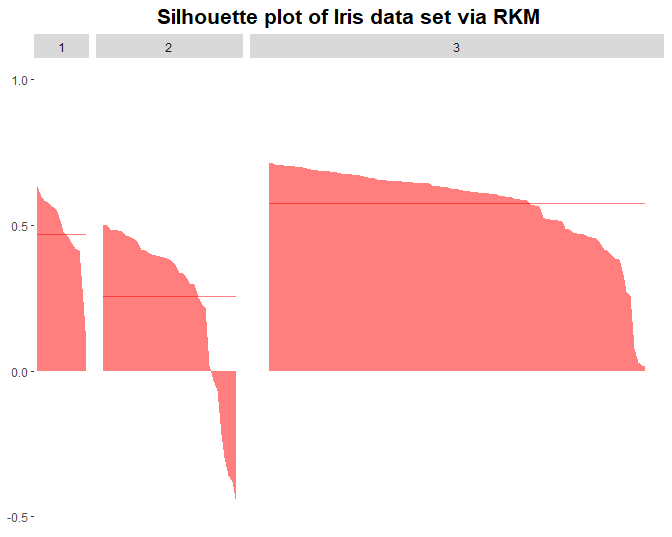(Back to Intoduction)

### 4.A.2. Centroid-based shadow value (csv)

An other way to measure internal validation with its corresponding plot is by presenting the centroid-based shadow value (Leisch 2010). The csv function calculates and plots the centroid-base shadow value of each object, which is based on the first and second closest medoids. The centroid of the original version of the csv is replaced by medoids in the csv function to adapt the k-medoids algorithm.

The required arguments in the csv function is identical to the silhouette (sil) function. Thus, the shadow value and plot of the best clustering result of iris data set via RKM can be obtained by

#calculate centroid-base shadow value of the RKM result of iris data set
csviris <- csv(mrwdist, rkm$medoid, rkm$cluster,
title = "CSV plot of Iris data set via RKM")

The centroid-based shadow values of objects 49 to 52, for instance, are presented by

#shadow values of objects 49 to 52
csviris$result[c(49:52),] ## csv cluster ## 49 0.7899687 2 ## 50 0.0000000 2 ## 51 0.3604380 3 ## 52 0.2473829 3 The centroid-based shadow value plot is also produced. csviris$plot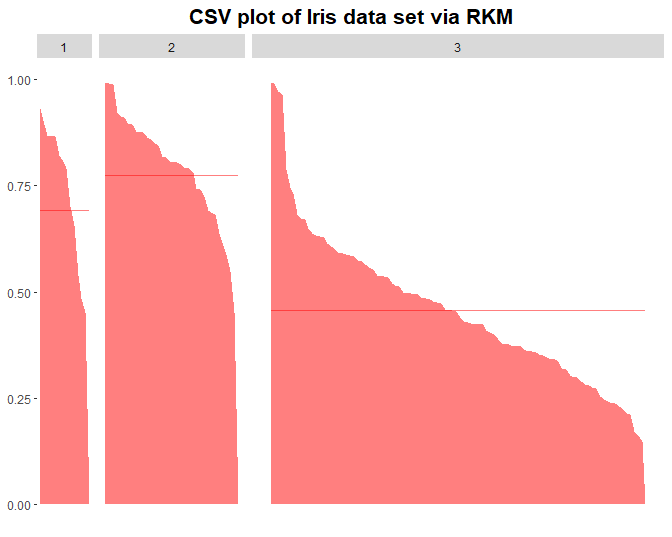(Back to Intoduction)

### 4.A.3. Medoid-based shadow value (msv)

An other way to measure internal validation by combining silhoutte and csv properties is by a medoid-based shadow value (msv) (Budiaji 2019). The msv function calculates and plots the medoid-based shadow value of each object, which is based on the first and second closest medoids.

The required arguments in the msv function is identical to the centroid-based shadow value (csv) function. Thus, the medoid-based shadow value and plot of the best clustering result of iris data set via RKM can be obtained by

#calculate medoid-based shadow value of the RKM result of iris data set
msviris <- msv(mrwdist, rkm$medoid, rkm$cluster,
title = "MSV plot of Iris data set via RKM")

The medoid-based shadow values of objects 49 to 52, for instance, are presented by

#Medoid-based shadow values of objects 49 to 52
msviris$result[c(49:52),] ## msv cluster ## 49 0.3471503 2 ## 50 1.0000000 2 ## 51 0.7801620 3 ## 52 0.8588494 3 The shadow value plot is also produced. msviris$plot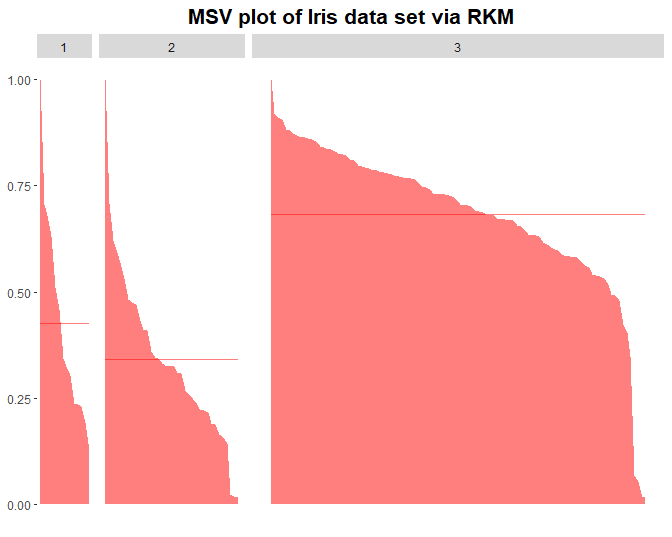(Back to Intoduction)

### 4.B. Relative criteria

The relative criteria evaluate a clustering algorithm result by applying re-sampling strategy. Thus, a bootstrap strategy can be applied. It is expected that the result of the cluster bootstraping is robust over all replications (Dolnicar and Leisch 2010). There are three steps to validate the cluster result via the boostraping strategy.

#### Step 1 Creating a matrix of bootstrap replicates

To create a matrix of bootstrap replicates, the clustboot function can be applied. There are five arguments in the clustboot function with the algorithm argument being the most important. The algorithm argument is the argument for a clustering algorithm that a user wants to evaluate. It has to be a function. When the RKM of iris data set is validated, for instance, the RKM function, which is required as an input in the algorithm argument, is

#The RKM function for an argument input
rkmfunc <- function(x, nclust) {
res <- rankkmed(x, nclust, m = 10, iterate = 50)
return(res$cluster) } When a function is created, it has to have two input arguments. They are x (a distance matrix) and nclust (a number of clusters). The output, on the other hand, is a vector of cluster membership (res$cluster). Thus, the matrix of bootstrap replicates can be produced by

#The RKM algorthim evaluation by inputing the rkmfunc function
#in the algorithm argument
rkmbootstrap <- clustboot(mrwdist, nclust=3, nboot=50, algorithm = rkmfunc)

with the objects 1 to 4 on the first to fifth replications being

rkmbootstrap[1:4,1:5]
##      [,1] [,2] [,3] [,4] [,5]
## [1,]    1    1    1    0    1
## [2,]    1    1    1    1    0
## [3,]    0    1    0    1    0
## [4,]    0    1    0    0    2

The rkmbootstrap is a matrix of bootrstrap replicates with a dimension of 150 x 50, i.e. n x b, where n is the number of objects and b is the number of bootstrap replicates. Note that the default evaluated algorithm is the SFKM algorithm such that if a user ignores the algorithm argument, the matrix of bootstrap replicates can still be produced. However, it misleads because it does not evaluate the user’s own algorithm.

#### Step 2 Transforming the bootstrap matrix into a consensus matrix

The matrix of bootstrap replicates produced by the clustboot in the step 1 can be transformed into a consensus matrix with a dimension of n x n via the consensusmatrix function. An element of the consensus matrix in row i dan column j is an agreement value between objects i and j to be in the same cluster when they are taken as a sample at the same time (Monti et al. 2003).

However, it requires an algorithm to order the objects in such a way that objects in the same cluster are close to each other. The consensusmatrix function has the reorder argument to comply this task. It is similar to the algorithm argument in the clustboot function in the step 1 where the reorder has to be a function that has two arguments and a vector of output.

Transforming the rkmbootstrap into a consensus matrix via the ward linkage algorithm to oder the objects, for example, can obtained by

#The ward function to order the objects in the consensus matrix
wardorder <- function(x, nclust) {
res <- hclust(as.dist(x), method = "ward.D2")
member <- cutree(res, nclust)
return(member)
}
consensusrkm <- consensusmatrix(rkmbootstrap, nclust = 3, wardorder)

The first to fourth rows and columns can be displayed as

consensusrkm[c(1:4),c(1:4)]
##           1         1         1         1
## 1 1.0000000 0.8823529 0.7333333 0.8421053
## 1 0.8823529 1.0000000 1.0000000 0.9444444
## 1 0.7333333 1.0000000 1.0000000 0.9473684
## 1 0.8421053 0.9444444 0.9473684 1.0000000

#### Step 3 Visualizing the consensus matrix in a heatmap

The ordered consensus matrix in the step 2 can be visualized in a heatmap applying the clustheatmap function. The agreement indices in the consensus matrix can be transformed via a non-linear transformation (Hahsler and Hornik 2011). Thus, the consensusrkm can visualize into

clustheatmap(consensusrkm, "Iris data evaluated by the RKM, ordered by Ward linkage")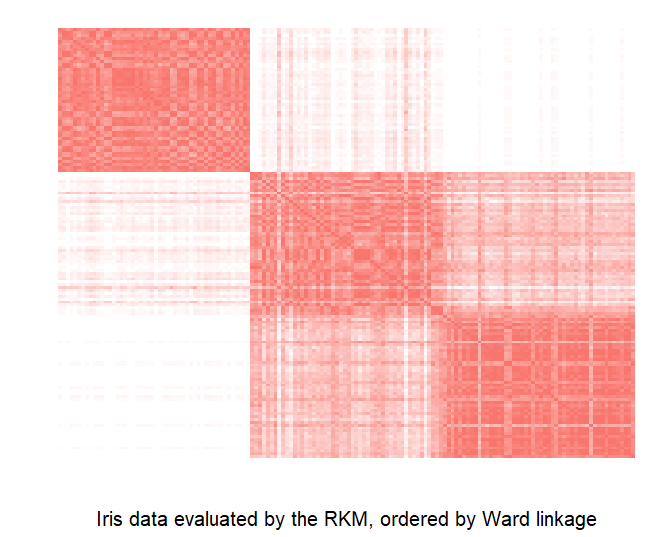(Back to Intoduction)

## 5. Cluster visualization

A cluster visualization of the clustering result can enhance the data structure understanding. The biplot and marked barplot are presented to visualize the clustering result.

### A. Biplot

The pcabiplot function can be applied to plot a clustering result from a numerical data set. The numerical data set has to be converted into a principle component object via the prcomp function. The x and y axes in the plot can be replaced by any component of the principle components. The colour of the objects can be adjusted based on the cluster membership by supplying a vector of membership in the colobj argument.

The iris data set can be plotted in a pca biplot with the colour objects based on the RKM algorithm result.

#convert the data set into principle component object
pcadat <- prcomp(iris[,1:4], scale. = TRUE)
#plot the pca with the corresponding RKM clustering result
pcabiplot(pcadat, colobj = rkm$cluster, o.size = 2)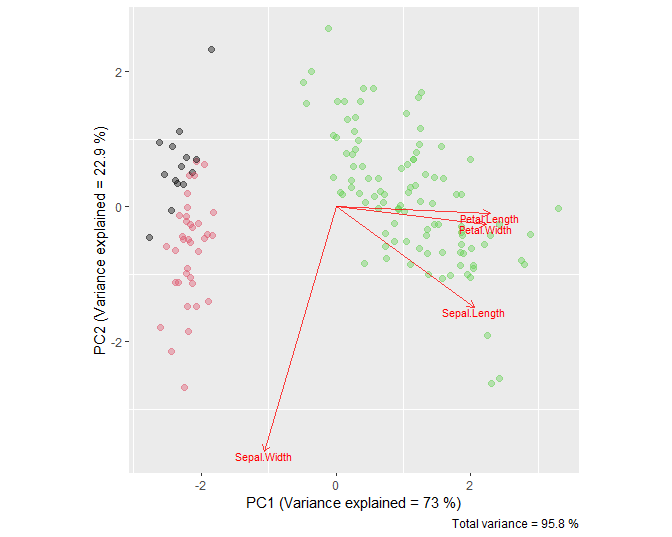The second principle component can be replaced by the third principle component. pcabiplot(pcadat, y = "PC3",colobj = rkm$cluster, o.size = 1.5)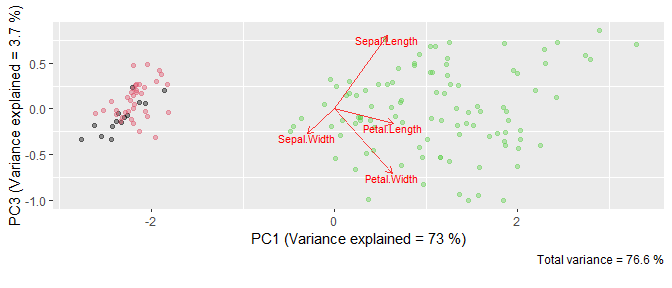(Back to Intoduction)

### B. Marked barplot

A marked barplot has been proposed by Dolnicar and Leisch (2014); Leisch (2008) where the mark indicates a significant difference between the cluster’s mean and population’s mean in each variable. The barplot function creates a barplot of each cluster with a particular significant level. The layout of the barplot is set in the nc argument.

The barplot of iris data set partitioned by the RKM algorithm is

barplotnum(iris[,1:4], rkm$cluster, alpha = 0.05)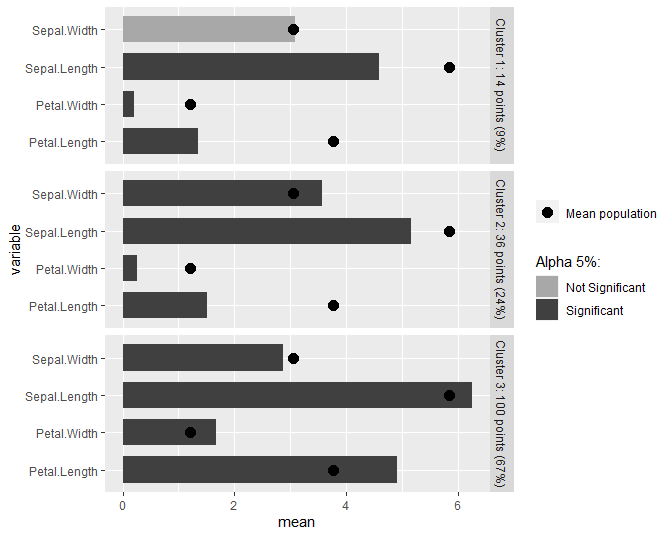while the layout is changed into 2 columns and the alpha is set into 1%, it becomes barplotnum(iris[,1:4], rkm$cluster, nc = 2, alpha = 0.01)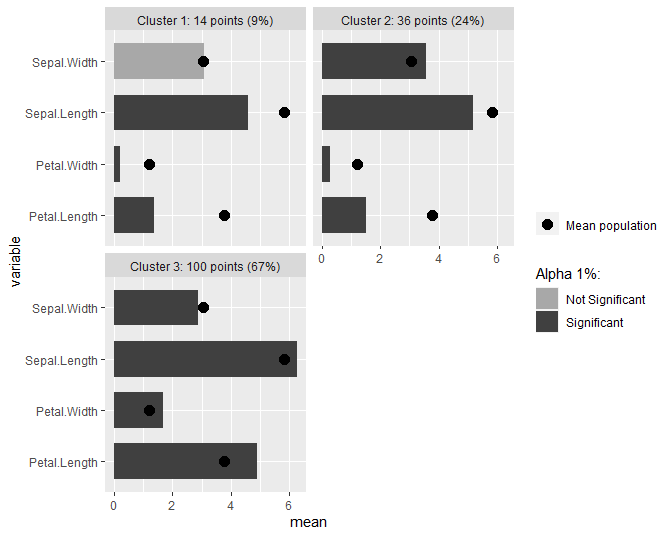(Back to Intoduction)

# References

Ahmad, A., and L. Dey. 2007. “A K-Mean Clustering Algorithm for Mixed Numeric and Categorical Data.” Data and Knowledge Engineering 63 (November): 503–27. https://doi.org/10.1016/j.datak.2007.03.016.
Budiaji, W. 2019. “Medoid-Based Shadow Value Validation and Visualization.” International Journal of Advances in Intelligent Informatics 5 (July): 76–88. https://doi.org/10.26555/ijain.v5i2.326.
Budiaji, W., and F. Leisch. 2019. “Simple K-Medoids Partitioning Algorithm for Mixed Variable Data.” Algorithms 12 (August): 177. https://doi.org/10.3390/a12090177.
Dolnicar, S., and F. Leisch. 2010. “Evaluation of Structure and Reproducibility of Cluster Solutions Using the Bootstrap.” Marketing Letters 21 (March): 83–101.
———. 2014. “Using Graphical Statistics to Better Understand Market Segmentation Solutions.” International Journal of Market Research 56 (March): 207–30.
Gower, J. 1971. “A General Coefficient of Similarity and Some of Its Properties.” Biometrics 27 (December): 857–71.
Hahsler, M., and K. Hornik. 2011. “Consensus Clustering; Dissimilarity Plots; A Visual Exploration Tool for Partitional Clustering.” Journal of Computational and Graphical Statistics 20 (August): 335–54. https://doi.org/10.1198/jcgs.2010.09139.
Harikumar, S., and S. PV. 2015. “K-Medoid Clustering for Heterogeneous Data Sets.” JProcedia Computer Science 70: 226–37. https://doi.org/10.1016/j.procs.2015.10.077.
Huang, Z. 1997. “Clustering Large Data Sets with Mixed Numeric and Categorical Values.” In The First Pacific-Asia Conference on Knowledge Discovery and Data Mining, 21–34.
Leisch, F. 2008. “Visualizing Cluster Analysis and Finite Mixture Models.” In Handbook of Data Visualization, 561–87. Springer Verlag.
———. 2010. “Neighborhood Graphs, Stripes and Shadow Plots for Cluster Visualization.” Statistics and Computing 20 (October): 457–69.
Monti, S., P. Tamayo, J. Mesirov, and T. Golub. 2003. “Consensus Clustering; A Resampling-Based Method for Class Discovery and Visualization of Gene Expression Microarray Data.” Machine Learning 52 (July): 91–118.
Park, H., and C. Jun. 2009. “A Simple and Fast Algorithm for K-Medoids Clustering.” Expert Systems with Applications 36 (2): 3336–41. https://doi.org/10.1016/j.eswa.2008.01.039.
Podani, J. 1999. “Extending Gower’s General Coefficient of Similarity to Ordinal Characters.” Taxon 48 (May): 331–40.
Reynolds, A. P., G. Richards, B. De La Iglesia, and V. J. Rayward-Smith. 2006. “Clustering Rules; A Comparison of Partitioning and Hierarchical Clustering Algorithms.” Journal of Mathematical Modelling and Algorithms 5 (March): 475–504.
Rousseeuw, P. J. 1987. “A Graphical Aid to the Interpretation and Validation of Cluster Analysis.” Journal of Computational and Applied Mathematics 20 (November): 53–65. https://doi.org/10.1016/0377-0427(87)90125-7.
Wishart, D. 2003. “K-Means Clustering with Outlier Detection, Mixed Variables and Missing Values.” In Exploratory Data Analysis in Empirical Research; Proceedings of the 25th Annual Conference of the Gesellschaft Fur Klassifikation e.v., University of Munich, March 14-16, 2001, 27:216–26. Springer Berlin Heidelberg.
Yu, D., G. Liu, M. Guo, and X. Liu. 2018. “An Improved K-Medoids Algorithm Based on Step Increasing and Optimizing Medoids.” Expert Systems with Applications 92 (February): 464–73. https://doi.org/10.1016/j.eswa.2017.09.052.
Zadegan, S.M.R, M. Mirzaie, and F. Sadoughi. 2013. “Ranked k-Medoids A Fast and Accurate Rank-Based Partitioning Algorithm for Clustering Large Datasets.” Knowledge-Based Systems 39 (February): 133–43. https://doi.org/10.1016/j.knosys.2012.10.012.